# JP5652434B2 - Motor control device and electric power steering device using the same - Google Patents

Motor control device and electric power steering device using the same Download PDF

## Info

Publication number
JP5652434B2
JP5652434B2 JP2012136200A JP2012136200A JP5652434B2 JP 5652434 B2 JP5652434 B2 JP 5652434B2 JP 2012136200 A JP2012136200 A JP 2012136200A JP 2012136200 A JP2012136200 A JP 2012136200A JP 5652434 B2 JP5652434 B2 JP 5652434B2
Authority
JP
Japan
Prior art keywords
phase
calculation
motor
control
value
Prior art date
Legal status (The legal status is an assumption and is not a legal conclusion. Google has not performed a legal analysis and makes no representation as to the accuracy of the status listed.)
Active
Application number
JP2012136200A
Other languages
Japanese (ja)
Other versions
JP2014003765A (en
Inventor

Original Assignee

Priority date (The priority date is an assumption and is not a legal conclusion. Google has not performed a legal analysis and makes no representation as to the accuracy of the date listed.)
Filing date
Publication date
Application filed by 株式会社デンソー filed Critical 株式会社デンソー
Priority to JP2012136200A priority Critical patent/JP5652434B2/en
Publication of JP2014003765A publication Critical patent/JP2014003765A/en
Application granted granted Critical
Publication of JP5652434B2 publication Critical patent/JP5652434B2/en
Active legal-status Critical Current
Anticipated expiration legal-status Critical

• 244000171263 Ribes grossularia Species 0.000 claims description 150
• 238000006243 chemical reactions Methods 0.000 claims description 61
• 238000000034 methods Methods 0.000 claims description 55
• 238000004804 winding Methods 0.000 claims description 17
• 230000005540 biological transmission Effects 0.000 claims description 2
• 101710044309 TROSPA Proteins 0.000 description 38
• 101710023022 stlA Proteins 0.000 description 38
• 230000014509 gene expression Effects 0.000 description 16
• 238000010586 diagrams Methods 0.000 description 13
• 238000004891 communication Methods 0.000 description 9
• 230000000875 corresponding Effects 0.000 description 9
• 239000011651 chromium Substances 0.000 description 5
• 230000002159 abnormal effects Effects 0.000 description 2
• 230000003321 amplification Effects 0.000 description 2
• 239000003990 capacitor Substances 0.000 description 2
• 238000003199 nucleic acid amplification method Methods 0.000 description 2
• 241001442055 Vipera berus Species 0.000 description 1
• 239000000969 carriers Substances 0.000 description 1
• 230000001808 coupling Effects 0.000 description 1
• 238000010168 coupling process Methods 0.000 description 1
• 238000005859 coupling reactions Methods 0.000 description 1
• 230000001186 cumulative Effects 0.000 description 1
• 238000001514 detection method Methods 0.000 description 1
• 238000006073 displacement reactions Methods 0.000 description 1
• 230000000694 effects Effects 0.000 description 1
• 238000005516 engineering processes Methods 0.000 description 1
• 238000007689 inspection Methods 0.000 description 1
• 238000009434 installation Methods 0.000 description 1
• 229910044991 metal oxides Inorganic materials 0.000 description 1
• 150000004706 metal oxides Chemical class 0.000 description 1
• 230000000051 modifying Effects 0.000 description 1
• 239000004065 semiconductors Substances 0.000 description 1
• 230000001502 supplementation Effects 0.000 description 1

## Images

•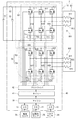••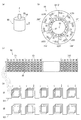••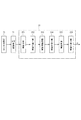••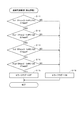••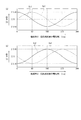•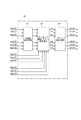•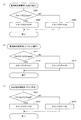•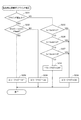•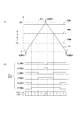•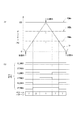•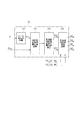•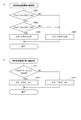•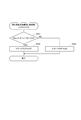•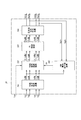•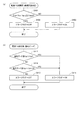•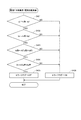•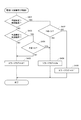•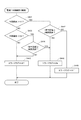•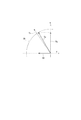•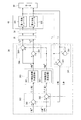•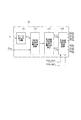•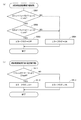•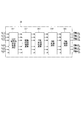•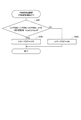••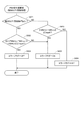## Classifications

• HELECTRICITY
• H02GENERATION; CONVERSION OR DISTRIBUTION OF ELECTRIC POWER
• H02PCONTROL OR REGULATION OF ELECTRIC MOTORS, ELECTRIC GENERATORS OR DYNAMO-ELECTRIC CONVERTERS; CONTROLLING TRANSFORMERS, REACTORS OR CHOKE COILS
• H02P27/00Arrangements or methods for the control of AC motors characterised by the kind of supply voltage
• H02P27/04Arrangements or methods for the control of AC motors characterised by the kind of supply voltage using variable-frequency supply voltage, e.g. inverter or converter supply voltage
• H02P27/06Arrangements or methods for the control of AC motors characterised by the kind of supply voltage using variable-frequency supply voltage, e.g. inverter or converter supply voltage using dc to ac converters or inverters
• BPERFORMING OPERATIONS; TRANSPORTING
• B62LAND VEHICLES FOR TRAVELLING OTHERWISE THAN ON RAILS
• B62DMOTOR VEHICLES; TRAILERS
• B62D5/00Power-assisted or power-driven steering
• B62D5/04Power-assisted or power-driven steering electrical, e.g. using an electric servo-motor connected to, or forming part of, the steering gear
• B62D5/0457Power-assisted or power-driven steering electrical, e.g. using an electric servo-motor connected to, or forming part of, the steering gear characterised by control features of the drive means as such
• B62D5/0481Power-assisted or power-driven steering electrical, e.g. using an electric servo-motor connected to, or forming part of, the steering gear characterised by control features of the drive means as such monitoring the steering system, e.g. failures
• BPERFORMING OPERATIONS; TRANSPORTING
• B62LAND VEHICLES FOR TRAVELLING OTHERWISE THAN ON RAILS
• B62DMOTOR VEHICLES; TRAILERS
• B62D5/00Power-assisted or power-driven steering
• B62D5/04Power-assisted or power-driven steering electrical, e.g. using an electric servo-motor connected to, or forming part of, the steering gear
• B62D5/0457Power-assisted or power-driven steering electrical, e.g. using an electric servo-motor connected to, or forming part of, the steering gear characterised by control features of the drive means as such
• B62D5/0481Power-assisted or power-driven steering electrical, e.g. using an electric servo-motor connected to, or forming part of, the steering gear characterised by control features of the drive means as such monitoring the steering system, e.g. failures
• B62D5/0493Power-assisted or power-driven steering electrical, e.g. using an electric servo-motor connected to, or forming part of, the steering gear characterised by control features of the drive means as such monitoring the steering system, e.g. failures detecting processor errors, e.g. plausibility of steering direction
• HELECTRICITY
• H02GENERATION; CONVERSION OR DISTRIBUTION OF ELECTRIC POWER
• H02PCONTROL OR REGULATION OF ELECTRIC MOTORS, ELECTRIC GENERATORS OR DYNAMO-ELECTRIC CONVERTERS; CONTROLLING TRANSFORMERS, REACTORS OR CHOKE COILS
• H02P21/00Arrangements or methods for the control of electric machines by vector control, e.g. by control of field orientation
• HELECTRICITY
• H02GENERATION; CONVERSION OR DISTRIBUTION OF ELECTRIC POWER
• H02PCONTROL OR REGULATION OF ELECTRIC MOTORS, ELECTRIC GENERATORS OR DYNAMO-ELECTRIC CONVERTERS; CONTROLLING TRANSFORMERS, REACTORS OR CHOKE COILS
• H02P21/00Arrangements or methods for the control of electric machines by vector control, e.g. by control of field orientation
• H02P21/13Observer control, e.g. using Luenberger observers or Kalman filters
• HELECTRICITY
• H02GENERATION; CONVERSION OR DISTRIBUTION OF ELECTRIC POWER
• H02PCONTROL OR REGULATION OF ELECTRIC MOTORS, ELECTRIC GENERATORS OR DYNAMO-ELECTRIC CONVERTERS; CONTROLLING TRANSFORMERS, REACTORS OR CHOKE COILS
• H02P25/00Arrangements or methods for the control of AC motors characterised by the kind of AC motor or by structural details
• H02P25/16Arrangements or methods for the control of AC motors characterised by the kind of AC motor or by structural details characterised by the circuit arrangement or by the kind of wiring
• H02P25/22Multiple windings; Windings for more than three phases
• HELECTRICITY
• H02GENERATION; CONVERSION OR DISTRIBUTION OF ELECTRIC POWER
• H02PCONTROL OR REGULATION OF ELECTRIC MOTORS, ELECTRIC GENERATORS OR DYNAMO-ELECTRIC CONVERTERS; CONTROLLING TRANSFORMERS, REACTORS OR CHOKE COILS
• H02P27/00Arrangements or methods for the control of AC motors characterised by the kind of supply voltage
• H02P27/04Arrangements or methods for the control of AC motors characterised by the kind of supply voltage using variable-frequency supply voltage, e.g. inverter or converter supply voltage
• H02P27/06Arrangements or methods for the control of AC motors characterised by the kind of supply voltage using variable-frequency supply voltage, e.g. inverter or converter supply voltage using dc to ac converters or inverters
• H02P27/08Arrangements or methods for the control of AC motors characterised by the kind of supply voltage using variable-frequency supply voltage, e.g. inverter or converter supply voltage using dc to ac converters or inverters with pulse width modulation
• HELECTRICITY
• H02GENERATION; CONVERSION OR DISTRIBUTION OF ELECTRIC POWER
• H02PCONTROL OR REGULATION OF ELECTRIC MOTORS, ELECTRIC GENERATORS OR DYNAMO-ELECTRIC CONVERTERS; CONTROLLING TRANSFORMERS, REACTORS OR CHOKE COILS
• H02P29/00Arrangements for regulating or controlling electric motors, appropriate for both AC and DC motors
• H02P29/02Providing protection against overload without automatic interruption of supply
• H02P29/024Detecting a fault condition, e.g. short circuit, locked rotor, open circuit or loss of load
• H02P29/0241Detecting a fault condition, e.g. short circuit, locked rotor, open circuit or loss of load the fault being an overvoltage

## Description

The present invention relates to a motor control device that controls driving of a motor, and an electric power steering device using the same.

Conventionally, when a failure has occurred in an inverter, a power relay, etc. that constitute a motor control device, or a failure has occurred in a current sensor or rotation angle sensor that detects each physical quantity input to the motor control device, these failures are detected. A technique for changing the control based on the fail-safe idea is known. However, the failure mode related to the motor control device is not limited to these hardware failures, and there may be a case where there is a bug in the control software executed by the microcomputer. Such software anomalies can have a significant impact on the user.

Therefore, for example, the apparatus disclosed in Patent Document 1 is provided with a hardware verification device separately from the microcomputer, and the verification device performs the same calculation and simple calculation in parallel independently of the microcomputer. By comparing the calculation output of the microcomputer and the inspection device, software abnormality of the microcomputer is detected.

Japanese Patent No. 4496205

The device of Patent Document 1 requires hardware different from the microcomputer. In addition, since the signal is output from the microcomputer to the hardware via the communication line, if the number of outputs becomes enormous due to the combination of monitoring targets, the monitoring range must be narrowed to avoid false detection. There's a problem. In addition, communication speed is restricted by the communication line.

The present invention has been made in view of the above-described problems, and an object of the present invention is to provide a motor control device capable of early detection of microcomputer software abnormality within a wide monitoring range without using separate hardware. There is.

The present invention uses a driving unit that drives a three-phase motor, an A / D converter that converts an analog electric signal from a sensor related to driving the motor into a digital electric signal, and an input signal from the A / D converter. In a motor control device comprising a microcomputer that calculates a control amount related to driving of the motor by executing control software and outputs a calculation result to the drive unit, the microcomputer controls the control in parallel with the control calculation. A “software monitoring process” for monitoring whether or not the calculation is normally executed is executed.
Here, the control calculation is a three-phase two-phase conversion calculation that dq-converts a three-phase current detection value (Iu, Iv, Iw) into a dq-axis current detection value (Id, Iq) using the electrical angle of the motor, and It includes a two-phase three-phase conversion operation for converting a two-phase voltage command value (Vd * , Vq * ) into a three-phase voltage command value (Vu * , Vv * , Vw * ) using the electrical angle of the motor .
In the software monitoring process, the microcomputer compares the value obtained by performing the two-phase / three-phase conversion on the dq-axis current detection value by the inverse dq conversion with the three-phase current detection value to determine whether the three-phase / two-phase conversion calculation is normal. Whether or not the two-phase / three-phase conversion calculation is normal is monitored by comparing a value obtained by performing three-phase / two-phase conversion of the three-phase voltage command value by dq conversion with a two-phase voltage command value.

Thereby, the motor control device of the present invention can detect the software abnormality of the microcomputer inside without using another hardware. Since no communication line is used, there is no need to provide a large margin in the monitoring area in consideration of the influence of noise and the like, and there is no restriction on the communication speed by the communication line. Therefore, the software abnormality of the microcomputer can be detected early in a wide monitoring range. Therefore, for example, it is effective when applied to a motor having a particularly high safety requirement such as a steering assist motor of an electric power steering apparatus.

By the way, if the microcomputer itself executes the software monitoring process together with the control calculation, it may be considered that the calculation load increases and consumes a lot of CPU resources. Regarding this point, for example, by setting the software monitoring cycle to a cycle longer than the control calculation cycle, it is possible to reduce the calculation load.

Further, it is preferable that the control software executed by the microcomputer is composed of a plurality of unit blocks that individually calculate the output from the input, and the software monitoring process is executed for each unit block. Thereby, since the presence or absence of the error in the relationship between an input and an output is monitored for every unit block, the calculation amount per monitoring unit can be reduced. Also, it is easy to identify an abnormal location. Furthermore, since the unit block monitoring can uniquely output the output compared with the whole block monitoring, the monitoring area can be set strictly.

The circuit schematic diagram of the motor control device by one embodiment of the present invention. 1 is a schematic configuration diagram of an electric power steering device to which a motor control device according to an embodiment of the present invention is applied. 1 is a schematic diagram of a motor to which a motor control device according to an embodiment of the present invention is applied. The control block diagram of the whole motor control apparatus by one Embodiment of this invention. The control block diagram of a mechanical angle calculating part. The flowchart of the software monitoring process of a mechanical angle calculating part. The flowchart of the software monitoring process of a mechanical angle calculating part. The schematic diagram which shows the structure of the detection circuit of a rotation angle sensor. Explanatory drawing which shows the output signal of the detection circuit of a rotation angle sensor. The control block diagram of an electric current detection calculating part. The flowchart of the software monitoring process of an electric current detection calculating part. The flowchart of the software monitoring process of an electric current detection calculating part. Explanatory drawing explaining the electric current detection timing in PWM control. Explanatory drawing explaining the blind correction by the upward shift process of a PWM command. The control block diagram of a 3 phase 2 phase conversion calculating part. The flowchart of the software monitoring process of a 3 phase 2 phase conversion calculating part. The flowchart of the software monitoring process of a 3 phase 2 phase conversion calculating part. The control block diagram of a current feedback calculating part. The flowchart of the software monitoring process of an electric current feedback calculating part. The flowchart of the software monitoring process of an electric current feedback calculating part. The flowchart of the software monitoring process of an electric current feedback calculating part. The flowchart of the software monitoring process of an electric current feedback calculating part. Explanatory drawing explaining the saturation guard of q-axis voltage command value. Explanatory drawing explaining an electric current sum difference calculation. The control block diagram of a 2 phase 3 phase conversion calculating part. The flowchart of the software monitoring process of a 2 phase 3 phase conversion calculating part. The control block diagram of a PWM command calculating part. The flowchart of the software monitoring process of a PWM command calculating part. The flowchart of the software monitoring process of a PWM command calculating part. The flowchart of the software monitoring process of a PWM command calculating part.

Embodiments of a motor control device according to the present invention will be described below with reference to the drawings.
(One embodiment)
[overall structure]
An overall configuration of an embodiment in which a motor control device according to the present invention is applied to an electric power steering device for a vehicle will be described with reference to FIGS.
FIG. 2 shows a steering system 90 provided with the electric power steering apparatus 1. A steering shaft 92 connected to the handle 91 is provided with a torque sensor 94 for detecting steering torque. A pinion gear 96 is provided at the tip of the steering shaft 92, and the pinion gear 96 meshes with the rack shaft 97. A pair of wheels 98 are rotatably connected to both ends of the rack shaft 97 via tie rods or the like. The rotational motion of the steering shaft 92 is converted into linear motion of the rack shaft 97 by the pinion gear 96, and the pair of wheels 98 are steered at an angle corresponding to the linear motion displacement of the rack shaft 97.

The electric power steering apparatus 1 includes an actuator 2 that rotates a rotating shaft, and a reduction gear 89 as “power transmission means” that decelerates and transmits the rotation of the rotating shaft to the steering shaft 92. The actuator 2 includes a steering assist motor 80 and a motor control device 10. The motor 80 is driven by the control of the motor control device 10, generates a steering assist torque for assisting the steering of the handle 91, and transmits the steering assist torque to the steering shaft 92.
The motor 80 of this embodiment is a three-phase AC brushless motor. The rotation angle of the motor 80 is detected by the rotation angle sensor 75.

Specifically, as shown in FIG. 1, the motor 80 has two winding sets 801 and 802.
The first winding set 801 includes U, V, and W phase three-phase windings 811, 812, and 813, and the second winding set 802 includes U, V, and W phase three-phase windings 821, 822. 823.
The inverter 60 includes a first system inverter 601 provided corresponding to the first winding set 801 and a second system inverter 602 provided corresponding to the second winding set 802. Hereinafter, a unit of a combination of an inverter and a three-phase winding set corresponding to the inverter is referred to as “system”.

The motor control device 10 includes A / D converters 11, 12, and 13 that are input side hardware, a microcomputer (hereinafter referred to as “microcomputer”) 30 that is software, and a drive unit 40 that is output side hardware. It consists of and.
The A / D converters 11, 12, and 13 receive analog electrical signals from the rotation angle sensor 75, the current sensor 70, and the torque sensor 94, respectively, and convert them into digital electrical signals.
The microcomputer 30 executes control software using input signals of the A / D converters 11, 12, and 13 to calculate a control amount related to driving of the motor 80 and outputs a calculation result to the drive unit 40.

The drive unit 40 includes a timer 41, a pre-driver 41, a power relay 52, a capacitor 53, a first system inverter 601, a second system inverter 602, a current sensor 70, and the like.
The timer 41 generates a drive signal for the pre-driver 42 based on the PWM command from the microcomputer 30. The pre-driver 42 drives the inverters 601 and 602 by turning on / off the switching elements.

The power supply relay 52 can cut off the power supply to the inverters 601 and 602 from the battery 51 which is, for example, a 12V DC power supply.
The capacitor 53 is connected in parallel with the battery 51, stores electric charge, assists power supply to the inverters 601 and 602, and suppresses noise components such as surge current.

In the first system inverter 601, six switching elements 611 to 616 are bridge-connected so as to switch energization to the windings 811, 812, and 813 of the first winding set 801. The switching elements 611 to 616 of this embodiment are MOSFETs (metal oxide semiconductor field effect transistors). Hereinafter, the switching elements 611 to 616 are referred to as MOSs 611 to 616. Further, the high potential side MOSs 611, 612, and 613 are referred to as “upper MOS”, and the low potential side MOSs 614, 615, and 616 are referred to as “lower MOS”.

The drains of the upper MOSs 611, 612, and 613 are connected to the positive electrode side of the battery 51. Further, the sources of the upper MOSs 611, 612 and 613 are connected to the drains of the lower MOSs 614, 615 and 616. Connection points between the upper MOSs 611, 612, and 613 and the lower MOSs 614, 615, and 616 are connected to one ends of the windings 811, 812, and 813, respectively. The gates of the MOSs 611 to 616 are connected to the pre-driver 42 and are turned on / off by a drive signal of the pre-driver 42.

The current sensor 70 includes a current sensor 701 of the first system and a current sensor 702 of the second system, and detects the phase current that the inverters 601 and 602 supply to the winding sets 801 and 802 for each phase. The current sensors 701 and 702 of this embodiment are configured by shunt resistors. The shunt resistors 711, 712, and 713 constituting the first system current sensor 701 are connected between the sources of the lower MOSs 614, 615, and 616 and the negative side of the battery 51, and the first system U, V, and W phases. The phase current supplied to the windings 811, 812, and 813 is detected.
Regarding the second system inverter 602, the configurations of the switching elements (MOS) 621 to 626 and the shunt resistors 721, 722 and 723 are the same as those of the first system inverter 601.

A specific configuration of the motor 80 will be described with reference to FIG. As shown in FIG. 3A, in the motor 80, the rotor 83 rotates with respect to the stator 84 around the rotation axis.
In the three-phase AC brushless motor according to this embodiment, when m is a natural number, the number of coils of the stator 84 is (12 × m), and the number of poles of the permanent magnet 87 of the rotor 83 is (2 × m). In the example shown in FIG. 3, m = 5. Note that m may be a natural number other than 5.

FIG. 3B is a schematic diagram of the permanent magnet 87 and the stator 84 of the rotor 83 viewed from the thrust direction Z (see FIG. 3A). The permanent magnet 87 is provided with a total of 10 (= 2 × 5) poles, each having 5 N poles and 5 S poles alternately.
The stator coil is composed of 10 groups of 6 coil groups, that is, 60 (= 12 × 5) coils. In one coil group, coils U1, U2, V1, V2, W1, and W2 are arranged clockwise in this order. Also, five areas (area 0 to area 4) corresponding to two coil groups are defined. If the upper side of the figure is 0 ° and the angle is shown in the clockwise direction, the angular position of the base end of each area is (n × 72 °, n = 0 to 4).

3C is a development view of the stator 84 as viewed from the thrust direction Z, and FIG. 3D is a development view of the winding as viewed from the radial direction R (see FIG. 3A). As shown in FIG. 3D, for example, the winding forming the U1 coil is formed by winding one conductive wire in turn on the protruding portions 86 arranged every six.
Thus, taking the U phase as an example, the circumferential arrangement of the U2 coil 821 constituting the second winding set 802 is 30 ° relative to the U1 coil 811 constituting the first winding set 801. The positional relationship is advanced by a corresponding angle. Therefore, the phase of the three-phase alternating current supplied to the second winding set 802 can be advanced by 30 ° with respect to the phase of the three-phase alternating current supplied to the first winding set 801.

Next, a control block diagram of the entire motor control apparatus 10 is shown in FIG. The configuration shown in FIG. 4 is not limited to the case of two systems but can be applied to a motor control apparatus of one system.
In FIG. 4, the A / D converters 11, 12, 13 and the drive unit 40, which are hardware, are as described in FIG. On the other hand, for the software in the microcomputer 30, a series of control calculation processes are divided into a plurality of unit blocks 31 to 37. This unit block is a processing unit for calculating the output from the input individually.

Specifically, the microcomputer 30 includes, as unit blocks, a mechanical angle calculation unit 31, a current detection calculation unit 32, a three-phase two-phase conversion calculation unit 33, a current feedback calculation unit 34, a two-phase three-phase conversion calculation unit 35, and a PWM. A command calculation unit 36 and a current command value calculation unit 37 are included. These unit blocks can be divided into more detailed blocks.

The outline of each calculation unit will be described. The mechanical angle calculation unit 31 acquires the rotation angle of the motor detected by the rotation angle sensor 75 as the output voltage of the A / D converter 11 and calculates the mechanical angle ψ. Then, the mechanical angle ψ is output to the three-phase / two-phase conversion calculation unit 33 and the two-phase / three-phase conversion calculation unit 35.
The current detection calculation unit 32 acquires the phase current detected by the current sensor 70 as the output voltage of the A / D converter 12, and calculates the three-phase current detection values Iu, Iv, and Iw.
The three-phase / two-phase conversion calculation unit 33 converts the three-phase current detection values Iu, Iv, and Iw into dq-axis current detection values Id and Iq, and outputs them to the current feedback calculation unit 34.

The current command value calculation unit 37 acquires the steering torque detected by the torque sensor 94 as the output voltage of the A / D converter 13 and calculates the q-axis current command value Iq * . Then, the q-axis current command value Iq * is output to the current feedback calculation unit 34.
The current feedback calculation unit 34 includes the q-axis current command value Iq * acquired from the current command value calculation unit 37, the d-axis current command value Id * calculated internally, and the dq from the three-phase / two-phase conversion calculation unit 33. Based on the deviations from the detected shaft current values Id and Iq, voltage command values Vd * and Vq * are generated by PI control and output to the two-phase / three-phase conversion calculation unit 35.

The two-phase / three-phase conversion calculation unit 35 converts the voltage command values Vd * and Vq * into U-phase, V-phase, and W-phase three-phase voltage command values Vu * , Vv * , and Vw * to generate a PWM command calculation unit 36. Output to. The PWM command calculation unit 36 calculates a PWM command representing the duty (%).
This PWM command is output from the microcomputer 30 to the timer 41 of the drive unit 40, and a switching signal for the upper and lower MOSs of the inverter 60 is generated by a triangular wave comparison. Based on the switching signal, the pre-driver 42 drives the inverter 60.

In general, in a motor control device, hardware such as a short circuit or disconnection failure of the MOSs 611 to 616, 621 to 626, the power supply relay 52, etc. of the inverters 601 and 602, or a failure of the detection unit of the current sensor 70 or the rotation angle sensor 75 is used. For failure, various failure detection methods have been proposed. Further, in the case of a device having a high safety requirement such as an electric power steering device, in general, when a failure occurs, the control is always changed to the safe side by the fail-safe concept.

However, no attention has been paid to the abnormalities of the control software in the microcomputer 30, so-called bugs. On the other hand, the motor control device 10 of the present embodiment is characterized by monitoring an abnormality of the control software in the microcomputer 30 in parallel with the control calculation. Hereinafter, this process is referred to as “software monitoring process”. The “software monitoring process” is to improve the reliability of the electric power steering apparatus 1 to which the motor control apparatus 10 is applied by detecting a software abnormality that may have a significant influence on the user. The software abnormality may be an abnormality in which the calculated value sticks to the maximum value, the minimum value, or another value, or an abnormality in which the calculated value is a double value of the normal value or a value of (1/2).

In the present embodiment, the software monitoring process is executed for each unit block. Here, the software monitoring process may be executed in the same calculation cycle as the control calculation, or when the need to reduce the calculation load is given priority, the software monitoring process is set to a period longer than the control calculation period. Also good.
Hereinafter, specific examples of the software monitoring process for the six calculation units 31 to 36 excluding the current command value calculation unit 37 will be described with reference to FIGS.

In the following explanation, when distinguishing the control amounts such as current and voltage in the two systems for each system, numbers “1” and “2” indicating the first system or the second system are added to the end of the symbol, and the system When not distinguishing each, a symbol excluding the numbers “1” and “2” is used.
In the software monitoring process, “zero approximate value” is defined in consideration of detection noise and the like, and when the absolute value of the monitoring target amount is equal to or less than “zero approximate value”, it is regarded as substantially equal to “zero”. For example, “zero approximate voltage value Vapp0” can be set to 0.1V, “zero approximate current value Vapp0” can be set to 1A, and “zero approximate duty Dapp0” can be set to 1%.

[Mechanical angle calculation unit 31]
The software monitoring process of the mechanical angle calculation unit 31 will be described with reference to FIGS.
As shown in FIG. 5, the calculations performed by the mechanical angle calculation unit 31 include a difference calculation 311, a first correction calculation 312, a pseudo signal calculation 313, a second correction calculation 314, an angle calculation 315, and a third correction calculation 316. .
Here, as the detection element of the rotation angle sensor 75, for example, a magnetoresistive element (MR) is employed. The impedance of the magnetoresistive element is changed by a rotating magnetic field that changes in accordance with the rotation of the detected object. In the present embodiment, the electrical angle of the magnetoresistive element corresponds to the mechanical angle ψ. Also, as shown in FIG. 3, the motor 80 of the present embodiment has five magnetic pole pairs, so the mechanical angle ψ corresponds to (1/5) of the motor electrical angle θ.

FIG. 6 is a flowchart of the monitoring process for comparing the calculated mechanical angles in the two systems. In the following description of the flow chart, the symbol S indicates “step”. In S101, the angle difference between the mechanical angle ψ calculated in both the first system and the second system and the mechanical angle ψ calculated in either the first system or the second system is a predetermined angle threshold ψ 1. -2 Determine if it is less than th. If the angle difference is less than or equal to the threshold ψ 1-2 th, the error flag is turned off (S102), and if it is greater than the threshold ψ 1-2 th, the error flag is turned on (S103).

The flowchart of FIG. 7 monitors the output signal of the detection circuit of the rotation angle sensor disclosed in Japanese Patent Application Laid-Open No. 2011-99846. As shown in FIG. 8, the detection circuit includes four half bridge circuits 210, 220, 230, and 240, an amplification unit 25, and a microcomputer 30 that is a control unit.
In the first half bridge 210, two magnetoresistive elements 211 and 212 are connected in series between the power supply Vcc and the ground GND. The voltage of the power supply Vcc is 5V, for example.
Similarly, in the second half bridge 220, the third half bridge 230, and the fourth half bridge 240, two magnetoresistive elements 221, 222, 231, 232, 241, and 242 are connected in series between the power supply Vcc and the ground GND, respectively. It is connected.

The midpoints 215, 225, 235, 245 of the half bridges 210, 220, 230, 240 are connected to the plus side of the operational amplifiers 251 to 254 of the amplification unit 25. In addition, an offset voltage of 2.5 V is input to the negative side of the operational amplifiers 251 to 254.
The amplifying unit 25 amplifies and offsets the output signal output from the midpoints 215, 225, 235, and 245 of the half bridges 210, 220, 230, and 240 and outputs it to the microcomputer 30. As a result, output signals shown in FIGS. 9A and 9B are output to the microcomputer 30.

That is, the output signal based on the output of the first half bridge 210 is V x1 , the output signal based on the output of the second half bridge 220 is V y1 , the output signal based on the output of the third half bridge 230 is V x2 , and the fourth half. If the output signal based on the output of the bridge 240 is V y2 and the amplitude is B, each output signal is expressed by the following equations 1.1 to 1.4 in relation to the rotation angle ψ.
V x1 = B1 cos ψ + 2.5 + C1 Formula (1.1)
V y1 = B2sinψ + 2.5 + C2 (formula (1.2))
V x2 = −B3 cos ψ + 2.5 + C3 Formula (1.3)
V y2 = −B4sin ψ + 2.5 + C4 Formula (1.4)
Here, the rotation angle ψ corresponds to the electrical angle of the magnetoresistive element, that is, the mechanical angle ψ.

The microcomputer 30 calculates the difference between the expression 1.1 and the expression 1.3, the difference between the expression 1.2 and the expression 1.4, and erases the offset voltage value. The ratio is calculated, and the mechanical angle ψ is calculated based on the result.
(V y1 −V y2 ) / (V x1 −V x2 )
= 2B′sinψ / 2B′cosψ = tanψ Expression (1.5)

Here, when the offset voltage 2.5V correction value is represented by Voff and the gain correction value is represented by G, the equations 1.1 to 1.4 can be rewritten as the following equations 1.6 to 1.9.
| V x1 − (B1 cos ψ + Voff1) / G1 | = 0 Equation (1.6)
| V y1 − (B2sinψ + Voff2) / G2 | = 0 Expression (1.7)
| V x2 + (B3cosψ−Voff3) / G3 | = 0 Equation (1.8)
| V y2 + (B4sinψ−Voff4) / G4 | = 0 Expression (1.9)

If the calculation is normal, a conditional expression is established in which the right side of Expressions 1.6 to 1.9 is regarded as “zero approximate voltage value Vapp0” or less. Therefore, in the flowchart of FIG. 7, in S111 to S114, it is determined whether or not these four conditional expressions are satisfied, and if all are satisfied, the error flag is turned off (S115), and if any one is not satisfied, the error flag is turned on. (S116).

[Current detection calculation unit 32]
The software monitoring process of the current detection calculation unit 32 will be described with reference to FIGS.
As shown in FIG. 10, the calculations performed by the current detection calculation unit 32 include a peak reading voltage calculation 321, an offset correction / gain correction calculation 322, and a blind correction calculation 323. The current detection calculation unit 32 receives “peak reading current A / D value” (ItA / D) and “valley reading current A / D value” (IbA / D) of each phase of each system, A control current (Ictrl) is output.

In the present embodiment, the driving unit 40 generates a MOS on / off signal of the inverter 60 by PWM control based on triangular wave comparison. Here, the current detection timing in the PWM control will be described with reference to FIG. In PWM control, each phase PWM command PWMu, PWMv, PWMw and a carrier wave are compared to generate a MOS on / off signal. In the method employed in the present embodiment, the upper MOS is turned off and the corresponding lower MOS is turned on in a section in which the triangular wave Cr exceeds the respective phase PWM commands PWMu, PWMv, and PWMw. Further, in a section where the triangular wave Cr falls below each phase PWM command signal PWMu, PWMv, PWMw, the upper MOS is turned on and the corresponding lower MOS is turned off.

In the example shown in FIG. 13, the U-phase, V-phase, and W-phase are in order from the largest PWM command. For example, in the section KV1, the triangular wave Cr is located below the U-phase PWM command PWMu and above the V-phase PWM command PWMv command signal and the W-phase PWM command PWMw. Therefore, for the U phase, the upper MOS is turned on and the lower MOS is turned off. For the V phase and the W phase, the upper MOS is turned off and the lower MOS is turned on.
As described above, the on / off state of the MOS can be indicated by the voltage vector pattern (see, for example, Japanese Patent Laid-Open No. 2012-50252).

In this embodiment, shunt resistors 711, 712, 713, etc. are provided on the lower MOS 614, 615, 616 etc. side. Therefore, the “peaks” output from the A / D converter 12 at the “zero voltage vector V0 generation period” when all the three-phase upper MOSs are turned off and all the three-phase lower MOSs are turned on, that is, at the peak of the triangular wave Cr. By detecting “reading voltage”, “current flowing through the lower MOS” is calculated. This current is called “mountain reading current”.
In addition, the “zero voltage vector V7 generation period” in which the lower MOSs of all three phases are turned off and the upper MOSs of all three phases are turned on, that is, the “valley” output by the A / D converter 12 at the timing of the valley of the triangular wave Cr. By detecting “read voltage”, “valley read current” for correction is calculated.

By the way, as the shortest time for the shunt resistor to detect the current, about 5 μs is required in consideration of the ringing time and the dead time. Then, when the period of the triangular wave is 50 μs, the generation period of the zero voltage vector V0 at 90% duty is 5 μs. Therefore, when the duty exceeds 90%, the generation period of the zero voltage vector V0 is shorter than 5 μs. Become. Therefore, the shortest current detection time of the shunt resistor cannot be ensured.

Therefore, in such a case, the current is not detected during the generation period of the zero voltage vector V0, but the current is supplied during the “period in which the two-phase lower MOS is turned on and the other one-phase lower MOS is turned off”. A detection method is known (see, for example, Japanese Patent No. 4715677). In this method, a current flowing through a two-phase shunt resistor in which the lower MOS is turned on is detected, and a one-phase current in which the lower MOS is turned off is estimated by Kirchhoff's law expressed by the following equation 2.1. This corresponds to “blind correction”.
Iu + Iv + Iw = 0 Formula (2.1)

The blind correction is performed in the generation period of the voltage vector V1 when the phase for estimating the current is the U phase, is performed in the generation period of the voltage vector V3 in the case of the V phase, and is performed in the generation period of the voltage vector V5 in the case of the W phase. Is called. That is, it is performed during an odd voltage vector generation period.
Further, in order to ensure the odd voltage vector generation period as long as possible in the blind correction, an “upshift process” shown in FIG. 14 is performed. In the example shown in FIG. 14, the U-phase, V-phase, and W-phase are in order from the larger PWM command. In this case, the voltage average value is shifted upward to the high voltage side so that the maximum U-phase PWM command PWMu is 100%. Thereby, the zero voltage vector V0 generation period before the shift is eliminated, and the voltage vector V1 generation period before and after the zero voltage vector V0 generation period is a series of periods. As a result, current detection is performed during the voltage vector V1 generation period.

The flowchart in FIG. 11A monitors whether or not the peak reading voltage calculation 321 is normal. In S201, the average value Vta of the peak reading voltage is compared with the offset value 2.5V. If the absolute value of the voltage difference is equal to or less than the zero approximate voltage value Vapp0, the error flag is turned off (S202), and if it exceeds Vapp0, the error flag is turned on (S203).

The flowchart of FIG. 11B monitors whether the offset correction calculation 322 is normal. In S211, the valley reading voltage Vb is compared with the voltage value obtained by adding the offset value 2.5V to the voltage value Vo before gain correction. If the absolute value of the voltage difference is equal to or less than the zero approximate voltage value Vapp0, the error flag is turned off (S212), and if it exceeds Vapp0, the error flag is turned on (S213).

The flowchart in FIG. 11C monitors whether the gain correction calculation 322 is normal. In S221, the voltage value Vo before gain correction is compared with the value obtained by dividing the voltage value Vg after gain correction by a coefficient k. If the absolute value of the voltage difference is equal to or less than the zero approximate voltage value Vapp0, the error flag is turned off (S222), and if it exceeds Vapp0, the error flag is turned on (S223).

The flowchart of FIG. 12 monitors whether the blind correction calculation 323 is normal. When blind correction is being performed (S231: YES), Kirchhoff's law at the coupling point of the U, V, and W phases is premised. Therefore, if the calculation is normal, the sum of the three-phase control currents is “0”. ". Therefore, if the absolute value of the sum Sum (Iu, v, wctrl) of the three-phase control currents is less than or equal to the zero approximate current value Iapp0, the error flag is turned off (S233), and if it exceeds Iapp0, the error flag is turned on ( S234).

On the other hand, when the blind correction is not executed (S231: YES), if the calculation is normal, the product value of the detection currents Iu, Iv, Iw of each phase and the control currents Iuctrl, Ivctrl, Iwctrl becomes negative. It cannot be. Therefore, if all of S235, S236, and S237 are NO, the error flag is turned off (S238), and if any one of them is YES, the error flag is turned on (S239).

[Three-phase to two-phase conversion calculation unit 33]
The software monitoring process of the three-phase / two-phase conversion calculation unit 33 will be described with reference to FIGS.
As shown in FIG. 15, the calculation executed by the three-phase / two-phase conversion calculation unit 33 includes a motor electric angle calculation 331, a three-phase / two-phase conversion electric angle calculation 332, and each system three-phase / two-phase conversion electric angle calculation 333. , Each system three-phase two-phase conversion calculation 334 is included.

The motor electrical angle calculation 331 calculates the corrected motor electrical angle θa based on the corrected mechanical angle ψ output from the mechanical angle calculation unit 31. In the present embodiment, the corrected motor electrical angle θa corresponds to an angle five times the mechanical angle ψ.
The electric angle calculation 332 for three-phase two-phase conversion and the electric angle calculation 333 for three-phase two-phase conversion for each system are for three-phase two-phase conversion from the corrected motor electric angle θa and the motor rotation angular velocity ω LPF after the low-pass filter. The electrical angles θb1 and θb2 are calculated. Here, due to the configuration of the motor 80 shown in FIG. 3, the electrical angle θb2 of the second system advances by 30 ° with respect to the electrical angle θb1 of the first system. The difference in electrical angle of 30 ° is expressed by the following equation 3.1 using (θa ± 15 °).
θb2−θb1 = (θa + 15 °) − (θa−15 °) Expression (3.1)

The flowchart in FIG. 16A monitors the operation at this stage. If the calculation is normal, the absolute value of the angle difference between θb1 and (θa−15 °) for the first system and the angle difference between θb2 and (θa + 15 °) for the second system are both predetermined. Should be less than the angle threshold θth. Therefore, in S301, the angle difference between θb1 and (θa−15 °) is compared with the threshold θth, and in S302, the angle difference between θb2 and (θa + 15 °) is compared with the threshold θth. If the absolute values of the angle differences are both less than the threshold value θth, the error flag is turned off (S303), and if at least one system is greater than or equal to the threshold value θth, the error flag is turned on (S304).

Further, each system three-phase two-phase conversion calculation 334 performs dq conversion of the three-phase current detection values Iu, Iv, and Iw of each system into dq-axis current detection values Id and Iq using the electrical angles θb1 and θb2.
The flowchart of FIG. 16B monitors whether the three-phase / two-phase conversion calculation is normal. In S311, for each system, the values (Id, q2 → 3) obtained by performing two-phase three-phase conversion on the dq-axis current detection values Id, Iq by inverse dq conversion are compared with the three-phase current detection values Iu, Iv, Iw. If the absolute value of the current difference is equal to or less than the zero approximate current value Iapp0, the error flag is turned off (S312), and if it exceeds Iapp0, the error flag is turned on (S313).

Further, the flowchart of FIG. 17 monitors the consistency between the calculated electrical angle θ and mechanical angle ψ for each of the five areas of the motor 80. In S321, n corresponds to area numbers 0, 1, 2, 3, and 4. For each area, if the difference between (1/5) of the electrical angle θ and the mechanical angle (ψ + n × 72 °) relative to the base end of the area is less than the predetermined mechanical angle threshold ψth, the error flag is turned off. (S322), and if it is equal to or greater than the mechanical angle threshold ψth, the error flag is turned on (S323).

[Current feedback calculator 34]
The software monitoring process of the current feedback calculation unit 34 will be described with reference to FIGS. In the figure, “F / B” means “feedback”.
As shown in FIG. 18, the calculation performed by the current feedback calculation unit 34 includes a dq-axis current sum difference calculation 341, a dq-axis current deviation calculation 342, a PI control calculation 343, each system voltage command calculation 344, and a d-axis current command value. Computation 345 is included.

The current feedback calculation unit 34 receives the dq-axis current detection values Iq and Id from the three-phase / two-phase conversion calculation unit 33 and outputs the dq-axis voltage command values Vq * and Vd * to the two-phase / three-phase conversion calculation unit 35. . The flow of the entire control will be described later with reference to FIG. 24, and here, in particular, supplementation will be given regarding the generation of dq-axis current command values Iq * and Id * .
The q-axis current command value Iq * is generated by the current command value calculation unit 37 and input to the dq-axis current deviation calculation 342. On the other hand, the d-axis current command value Id * is generated by the d-axis current command value calculation 345 in the current feedback calculation unit 34 and input to the dq-axis current deviation calculation 342. The d-axis current command value calculation 345 is based on the “Iq sum” calculated by the dq-axis current sum difference calculation 341 and the q-axis voltage command values Vq1 * and Vq2 * of each system output by each system voltage command calculation 344. To calculate the d-axis current command value Id * .

The flowchart in FIG. 19A monitors the validity of the q-axis voltage command value Vq * of each system output by each system voltage command calculation 344. In S401, the value obtained by dividing the q-axis voltage command value Vq * by the voltage sensitive gain Gvs is compared with the feedback control q-axis voltage value Vq F / B. If the absolute value of the voltage difference is equal to or less than the zero approximate voltage value Vapp0, the error flag is turned off (S402), and if it exceeds Vapp0, the error flag is turned on (S403).

The flowchart of FIG. 19B monitors the validity of the q-axis voltage command value Vq * after saturation guard. Here, as shown in FIG. 23, the saturation guard refers to a process in which the q-axis voltage command value Vq * falls within the reference voltage circle CA defined by the following equation 4.1.
Vd 2 + Vq 2 = Va 2 (Formula 4.1)
That is, when the q-axis voltage command value Vq * is outside the reference voltage circle CA, it is corrected by the saturation guard so that it falls inside the reference voltage circle CA.

In S411 of FIG. 19B, q-axis voltage command values Vq * before and after saturation guard are compared. As described above, the saturation guard is a process for correcting the q-axis voltage command value Vq * to be inside the reference current circle CA. Therefore, if the calculation is normal, the q-axis voltage command value Vq * after saturation guard is performed . Cannot be larger than the original q-axis voltage command value Vq * (S411: YES). In addition, the product of the q-axis voltage command value Vq * before and after the saturation guard cannot be negative (S412: YES).
Therefore, if any of S411 and S412 is NO, the error flag is turned off (S413), and if either one is YES, the error flag is turned on (S414).

The flowchart of FIG. 20 monitors the current sum difference calculation of two systems. Here, a specific flow of the current sum difference calculation will be described with reference to FIG. In FIG. 24, for simplicity of explanation, the current and voltage are shown without being decomposed into dq-axis vectors.
As illustrated in FIG. 24, the current feedback calculation unit 34 controls “sum and difference of two systems of current” expressed by the following equations 4.2 and 4.3.
I sum = I1 + I2 (formula 4.2)
I difference = I1−I2 (formula 4.3)

First, for control of the current sum of the two systems, the current adder 381 of the current sum difference calculation 341 includes a detected value I1 of the output current of the first system inverter 601 and a detected value I2 of the output current of the second system inverter 602. And “I sum” is output.
The current sum deviation calculation unit 382 of the current deviation calculation 342 includes a deviation “E (I sum) between“ I sum * ”that is the sum of the current command values of the two systems and“ I sum ”that is the sum of the detected current values. Is calculated and output to the sum calculator 383 of the PI control calculation 343. The sum calculator 383 calculates “V sum * ” which is the sum of the voltage command values shown in the following equation 4.4 by proportional integral control calculation so that “E (I sum)” converges to zero.
V sum * = V1 * + V2 * Expression (4.4)

On the other hand, for the control of the current difference between the two systems, the current subtractor 391 of the current sum difference calculation 341 subtracts the detected value I2 of the output current of the second system inverter 602 from the detected value I1 of the output current of the first system inverter 601. And “I difference” is output.
The current difference calculation unit 392 of the current deviation calculation 342 includes a difference “E (I difference)” between the “I difference * ” that is the difference between the current command values of the two systems and the “I difference” that is the difference between the current detection values. Is calculated and output to the difference calculator 393 of the PI control calculation 343. The difference calculator 393 calculates “V difference * ”, which is the difference between the voltage command values shown in the following equation 4.5, by proportional integral control calculation so that “E (I difference)” converges to zero.
V difference * = V1 * −V2 * Expression (4.5)
Here, since the electrical characteristics of the first system inverter 601 and the second system inverter 602 are equivalent, the I difference * which is the difference between the current command values of the two systems is 0 (A) in principle.

For each system voltage command calculation 344, the first system voltage calculator 384 calculates the first system voltage command value V1 by the following equation 4.6. The voltage calculator 394 of the second system calculates the voltage command value V2 of the second system according to the following expression 4.7.
V1 * = (V sum * + V difference * ) / 2 Formula (4.6)
V2 * = (V sum * −V difference * ) / 2 Expression (4.7)
In the above equations 4.2 to 4.7, in the dq axis coordinate system, I is replaced with Id and Iq, and V is replaced with Vd and Vq.

In S421 to S424 of the flowchart of FIG. 20, it is monitored whether or not the current sum difference calculation is normal. In the flowchart of FIG. 20, the current and voltage are shown on the dq axis.
In S421, it is determined whether or not the q-axis current detection value Iq1 of the first system matches the value obtained by subtracting the second system q-axis current detection value Iq2 from the “Iq sum” that is the sum of the q-axis current detection values. To do.
In S422, it is determined whether or not the q-axis current detection value Iq1 of the first system matches the value obtained by adding the second system q-axis current detection value Iq2 to the “Iq difference” that is the difference between the q-axis current detection values. To do.

In S423, it is determined whether or not “Iq * sum”, which is the sum of the q-axis current command values, and a value obtained by adding “Iqsum” to the sum deviation “E (sum of Iq)”.
In S424, whether or not “Iq * difference” that is the difference between the q-axis current command values, that is, “0 * ”, and the difference deviation “E (Iq difference)” plus “Iq difference” match. Judge.
If all the conditions of S421 to S424 are satisfied, the error flag is turned off (S425), and if any one is NO, the error flag is turned on (S426).

The flowcharts of FIGS. 21 and 22 monitor the calculation related to the PI control calculation 343 of the current feedback control.
The flowchart of FIG. 21 relates to a constant of proportional control (P control). Proportional control is a control that gives an operation amount proportional to the deviation obtained by subtracting the current value from the target value. Therefore, if the calculation is normal, the current deviation in the monitoring cycle is larger than the previous deviation (S431: YES). Cannot be negative (S432: YES). On the other hand, when the current deviation is smaller than the previous deviation (S433: YES), the value of the P term cannot be positive (S434: YES).
Therefore, if NO in S432 and S434, the error flag is turned off (S435), and if either one is YES, the error flag is turned on (S436).

The flowchart of FIG. 22 relates to a constant of integral control (I control). Since the integral control is a control that gives an operation amount proportional to the cumulative value of the deviation so far, if the calculation is normal, if the current deviation in the monitoring period is undershoot greater than 0 A (S441: YES), I The term current value cannot be less than the previous value of I term (S442: YES). On the other hand, if the current deviation is an overshoot smaller than 0A (S443: YES), the current value of the I term cannot exceed the previous value of the I term (S444: YES).
Therefore, if NO in S442 and S444, the error flag is turned off (S445), and if either is YES, the error flag is turned on (S446).

[Two-phase / three-phase conversion calculation unit 35]
The software monitoring process of the two-phase / three-phase conversion calculation unit 35 will be described with reference to FIGS.
As shown in FIG. 25, the calculation executed by the two-phase / three-phase conversion calculation unit 35 includes a motor electric angle calculation 351, a two-phase / three-phase conversion electric angle calculation 352, and a two-phase / three-phase conversion electric angle calculation 353 for each system. Each system 2 phase 3 phase conversion calculation 354 is included.

The motor electrical angle calculation 351 is the same as the motor electrical angle calculation 331 of the three-phase / two-phase conversion calculation unit 33. Further, the two-phase / three-phase conversion electrical angle calculation 352 and each system two-phase / three-phase conversion electrical angle calculation 353 are calculated from the corrected motor electrical angle θa and the motor rotation angular velocity ω LPF after the low-pass filter. The electrical angles θc1 and θc2 for conversion are calculated. Here, the electrical angle θc2 of the second system is advanced by 30 ° with respect to the electrical angle θc1 of the first system. The difference in electrical angle of 30 ° is expressed by the following equation 5.1 using (θa ± 15 °).
θc2−θc1 = (θa + 15 °) − (θa−15 °) (formula 5.1)

The flowchart in FIG. 26 (a) monitors the operation at this stage. If the calculation is normal, the change in time T between the angle difference between θc1 and (θa−15 °) for the first system, and the angle difference between θc2 and (θa + 15 °) for the second system, and the motor rotation The absolute value of the difference from the angular velocity ω LPF should be less than the predetermined angular velocity threshold ωth. Therefore, the angular velocity difference of the first system is compared with the threshold ωth in S501, and the angular velocity difference of the second system in S502. If the absolute values of the angular velocity differences are both less than the threshold value ωth, the error flag is turned off (S503), and if at least one system is equal to or greater than the threshold value ωth, the error flag is turned on (S504).

Further, each system two- phase three- phase conversion calculation 354 converts the two-phase voltage command values Vd * and Vq * of each system into three-phase voltage command values Vu * , Vv * and Vw * using the electrical angles θc1 and θc2. To do.
The flowchart of FIG. 26B monitors whether the two-phase / three-phase conversion calculation is normal. In S511, for each system, the values (Vu, v, w * 3 → 2) obtained by converting the three-phase voltage command values Vu * , Vv * , Vw * by the three-phase dq conversion into the two-phase voltage command values Vd *. , Vq * . If the absolute value of the voltage difference is equal to or less than the zero approximate voltage value Vapp0, the error flag is turned off (S512), and if it exceeds Vapp0, the error flag is turned on (S513).

Note that the monitoring of the consistency between the calculated electrical angle θ and the mechanical angle ψ is the same as that in the flowchart of FIG.

[PWM command calculation unit 36]
The software monitoring process of the PWM command calculation unit 36 will be described with reference to FIGS.
As shown in FIG. 27, the calculations executed by the PWM command calculation unit 36 include a voltage utilization factor coefficient calculation calculation 361, a drive circuit compensation calculation 362, a PWM modulation calculation calculation 363, a PWM conversion calculation 364, and a PWM limit calculation 365.

The flowchart in FIG. 28 monitors whether the calculations up to the PWM conversion calculation 364 are normal. In S601, the symbol of the first term on the left side of the conditional expression has the following meaning.
PWMu−v * : (U phase PWM command−V phase PWM command) (%)
PWMv-w * : (V-phase PWM command-W-phase PWM command) (%)
PWMw-u * : (W-phase PWM command-U-phase PWM command) (%)
α: Coefficient for converting PWM command (%) to voltage (V /%)
That is, in S601, the voltage conversion value of the PWM command difference between each phase is converted into a three-phase two-phase conversion with the dq-axis voltage command values Vd * and Vq * . If the absolute value of the voltage difference is equal to or less than the zero approximate voltage value Vapp0, the error flag is turned off (S602), and if it exceeds Vapp0, the error flag is turned on (S603).

The flowchart in FIG. 29 monitors whether or not the PWM limit calculation 365 is normal. The PWM restriction restricts each phase PWM command so as to fall within a range from a predetermined lower limit value to an upper limit value. Therefore, if the calculation is normal, each phase PWM command (PWMu, v, w * ) ′ after the limit is in each phase before the limit in the region (S611: YES) above the intermediate value (50%) of the PWM command. It cannot be larger than the PWM command PWMu, v, w * (S612: YES). Further, in the region below the intermediate value (50%) of the PWM command (S613: YES), each phase PWM command (PWMu, v, w * ) ′ after the limit is changed to each phase PWM command PWMu, v, It cannot be smaller than w * (S614: YES).
Therefore, if NO in S612 and S614, the error flag is turned off (S615), and if either of them is YES, the error flag is turned on (S616).

The flowchart of FIG. 30 monitors whether the update calculation in the pseudo duty update process is normal. The pseudo-duty update process refers to a process of outputting a pseudo-duty multiple times in one cycle of the PWM command control calculation cycle in order to stabilize the motor control and reduce noise, vibration, torque ripple, and the like. For example, when the control calculation cycle is 200 μs, the pseudo duty is output at an update cycle of 100 μs corresponding to (1/2).

When the pseudo duty update process is performed, the pseudo PWM command is calculated by linear interpolation from, for example, the immediately preceding PWM command and the previous value of the PWM command one cycle before. Here, symbols are defined as follows.
PWMo (n): PWM command in the nth control calculation PWMo (n-1): PWM command in the (n-1) th control calculation (previous value)
PWMr (n): pseudo duty updated after 100 μs of the nth control calculation Then, the pseudo duty is calculated by the following equation 6.1.
PWMr (n) = {PWMo (n−1) + PWMo (n)} / 2 Formula (6.1)

Equation 6.1 is rewritten as Equation 6.2.
2 × PWMr (n) −PWMo (n−1) = PWMo (n) (formula 6.2)
On the other hand, when the pseudo duty update process is not performed, the output after 100 μs of the nth control calculation is equal to the PWM command in the nth control calculation as shown in the following equation 6.3.
PWMr (n) = PWMo (n) (formula 6.3)

According to the flowchart of FIG. 30, when the pseudo duty update process is performed (S621: YES), the error flag is turned off (S624) if the absolute value of the difference between both sides of Equation 6.2 is equal to or less than the zero approximate duty Dapp0. If it exceeds Dapp0, the error flag is turned on (S625). If the pseudo-duty update process is not performed (S621: NO), the error flag is turned off (S624) if the absolute value of the difference between both sides of Equation 6.3 is equal to or less than the zero approximate duty Dapp0, and if it exceeds Dapp0, an error occurs. The flag is turned on (S625).

[Summary]
As described above, the motor control device 10 according to the present embodiment allows the microcomputer 30 to perform software monitoring processing in parallel with the control calculation, so that the software abnormality of the microcomputer 30 can be prevented without using another monitoring hardware. Can be detected internally. In other words, since a communication line is not used as in the case of using another monitoring hardware, it is not necessary to provide a large margin for the monitoring threshold in order to avoid erroneous detection, and there is a restriction on the communication speed due to the communication line. Absent. Therefore, the software abnormality of the microcomputer 30 can be detected early in a wide monitoring range. Also, in the software monitoring process according to the present embodiment, the presence or absence of an error in the relationship between the input and the output is monitored for each unit block of the microcomputer 30. For this reason, the amount of calculation in the monitoring unit can be reduced. Also, it is easy to identify an abnormal location.

Furthermore, since the motor control device 10 of the present embodiment is applied to the two systems of the motor 80 and the drive unit 40, the calculation is normal by comparing the detection value or calculation value for each system between the two systems. Can be monitored. Therefore, the range of selection of a specific monitoring method in the software monitoring process is expanded.
In addition, the electric power steering device 1 to which the motor control device 10 of the present embodiment is applied is a device with particularly high safety requirements. Therefore, in addition to the detection of a hardware failure by the existing technology, the reliability can be further improved by executing the software monitoring process of the present embodiment.

The mechanical configuration of the motor of the above embodiment and the configuration of software related to motor control are merely examples. Therefore, the motor control device according to the present invention is not limited to the above embodiment, and can be implemented in various forms without departing from the spirit of the invention.
(A) The software monitoring process does not necessarily have to be executed for each unit block of the microcomputer. For example, it may be executed collectively for the control calculation of the entire microcomputer.
(A) The motor and the drive unit are not limited to two systems, and may be one system. In the case of two motors, the configuration is not limited to a configuration in which the phase difference between the systems is 30 °.
(C) The number of magnetic pole pairs of the motor is not limited to five. Depending on the number of magnetic pole pairs, the ratio between the motor electrical angle and the mechanical angle is appropriately changed.

(D) The method of the rotation angle sensor or current sensor is not limited to the above. When the shunt resistor is a current sensor, the installation location is not limited to the lower MOS side of the inverter but may be the upper MOS side, or may be between the inverter and the motor.
(E) The motor control device of the present invention is not limited to the steering assist motor of the electric power steering device, and may be applied as a control device for any other motor.

10: Motor control device,
11, 12, 13 ... A / D converter,
30 ... microcomputer (microcomputer),
70 ・ ・ ・ Current sensor (sensor),
75 ・ ・ ・ Rotation angle sensor (sensor),
80 ・ ・ ・ Motor,
94: Torque sensor (sensor).

## Claims (5)

1. A drive unit (40) for driving a three-phase motor (80);
An A / D converter (11, 12, 13) for converting an analog electric signal from a sensor (70, 75, 94) relating to driving of the motor into a digital electric signal;
A microcomputer (30) for calculating a control amount related to driving of the motor by executing control software using an input signal from the A / D converter, and outputting a calculation result to the driving unit;
With
The microcomputer executes a software monitoring process for monitoring whether the control calculation is normally executed in parallel with the control calculation ,
The control calculation includes a three-phase two-phase conversion calculation for dq-converting a three-phase current detection value (Iu, Iv, Iw) into a dq-axis current detection value (Id, Iq) using the electrical angle of the motor; and Including a two-phase three-phase conversion operation for converting a two-phase voltage command value (Vd * , Vq * ) into a three-phase voltage command value (Vu * , Vv * , Vw * ) using the electrical angle of the motor ;
In the software monitoring process, the microcomputer compares the three-phase current detection value with a value obtained by performing two-phase three-phase conversion on the dq-axis current detection value by inverse dq conversion, thereby performing the three-phase two-phase conversion calculation. Whether or not the two-phase three-phase conversion operation is normal is monitored by comparing whether the three-phase voltage command value is three-phase two-phase converted by dq conversion with the two-phase voltage command value. A motor control device (10) characterized by monitoring whether or not there is .
2. The control software executed by the microcomputer is composed of a plurality of unit blocks that individually calculate the output from the input,
The motor control apparatus according to claim 1, wherein the microcomputer executes the software monitoring process for each unit block.
3. The drive unit includes two inverters (601, 602) that are applied to a motor having two winding sets (801, 802) and supply electric power to the two winding sets. In the motor control device according to 2,
The microcomputer executes the software monitoring process by comparing a detection value detected for each system or a calculation value calculated based on the detection value between the systems. .
4. The software monitoring cycle in which the software monitoring process is executed is set to a cycle longer than the control operation cycle in which the control operation is executed. Motor control device.
5. A steering assist motor (80) for generating an assist torque for assisting the driver's steering;
The motor control device according to any one of claims 1 to 4 , which controls driving of the steering assist motor;
Power transmission means (89) for transmitting the torque of the steering assist motor to a steering shaft;
Electric power steering apparatus (1) provided with.
JP2012136200A 2012-06-15 2012-06-15 Motor control device and electric power steering device using the same Active JP5652434B2 (en)

## Priority Applications (1)

Application Number Priority Date Filing Date Title
JP2012136200A JP5652434B2 (en) 2012-06-15 2012-06-15 Motor control device and electric power steering device using the same

## Applications Claiming Priority (3)

Application Number Priority Date Filing Date Title
JP2012136200A JP5652434B2 (en) 2012-06-15 2012-06-15 Motor control device and electric power steering device using the same
US13/888,714 US8981687B2 (en) 2012-06-15 2013-05-07 Motor control apparatus and electric power steering apparatus using the same
CN201310236680.2A CN103507852B (en) 2012-06-15 2013-06-14 Controller for motor and the electrically powered steering apparatus using same using this controller for motor

## Publications (2)

Publication Number Publication Date
JP2014003765A JP2014003765A (en) 2014-01-09
JP5652434B2 true JP5652434B2 (en) 2015-01-14

# Family

## Family Applications (1)

Application Number Title Priority Date Filing Date
JP2012136200A Active JP5652434B2 (en) 2012-06-15 2012-06-15 Motor control device and electric power steering device using the same

## Country Status (3)

US (1) US8981687B2 (en)
JP (1) JP5652434B2 (en)
CN (1) CN103507852B (en)

## Families Citing this family (18)

* Cited by examiner, † Cited by third party
Publication number Priority date Publication date Assignee Title
US9531309B2 (en) * 2013-07-23 2016-12-27 Aisin Aw Co., Ltd. Drive device
JP5920300B2 (en) * 2013-09-18 2016-05-18 株式会社デンソー Power conversion device and electric power steering device using the same
JP5835301B2 (en) * 2013-10-22 2015-12-24 株式会社デンソー Power supply current monitoring device
WO2015125269A1 (en) * 2014-02-21 2015-08-27 三菱電機株式会社 Ac rotating machine control device and electric power steering control device
JP6015693B2 (en) * 2014-03-07 2016-10-26 株式会社デンソー Control device and electric power steering device using the same
US9712096B2 (en) 2014-04-28 2017-07-18 Mitsubishi Electric Corporation Control apparatus and control method for AC rotary machine, and electric power steering apparatus
WO2015182287A1 (en) * 2014-05-29 2015-12-03 日本精工株式会社 Electric power steering device
US10158315B2 (en) 2015-01-22 2018-12-18 Mitsubishi Electric Corporation Controller for AC rotating machine and controller for electric power steering
JP6250221B2 (en) * 2015-02-16 2017-12-20 三菱電機株式会社 Power converter
JP6250222B2 (en) * 2015-02-16 2017-12-20 三菱電機株式会社 Power converter
JP6540227B2 (en) * 2015-05-21 2019-07-10 株式会社ジェイテクト Vehicle control device
DE102015110174A1 (en) * 2015-06-24 2016-12-29 Carl Zeiss Industrielle Messtechnik Gmbh Method and device for testing an electrical variable of an electrical machine
JP6376067B2 (en) * 2015-07-27 2018-08-22 株式会社デンソー Control device and electric power steering device using the same
JP2017197137A (en) * 2016-04-28 2017-11-02 株式会社ジェイテクト Electric power steering device
JP6275211B2 (en) * 2016-07-29 2018-02-07 三菱電機株式会社 Electric power steering device
JP2018026905A (en) * 2016-08-08 2018-02-15 アイシン精機株式会社 Motor controller
JP6328280B1 (en) * 2017-01-25 2018-05-23 三菱電機株式会社 Control device for double winding type rotating electrical machine
JP2019161934A (en) * 2018-03-15 2019-09-19 トヨタ自動車株式会社 Motor controller, motor control program and motor control method

## Family Cites Families (16)

* Cited by examiner, † Cited by third party
Publication number Priority date Publication date Assignee Title
DE4192435C1 (en) 1990-10-03 2002-08-29 Hitachi Ltd Control for electric vehicle
JPH05103497A (en) * 1991-10-03 1993-04-23 Honda Motor Co Ltd Electric motor vehicle
JPH08123501A (en) 1994-10-27 1996-05-17 Toshiba Corp Process controller
JPH11155201A (en) 1997-11-20 1999-06-08 Hitachi Ltd Controller and control method for electric car
US6943512B2 (en) * 2002-02-04 2005-09-13 Nsk Ltd. Control apparatus for electric power steering system
CN2851056Y (en) * 2005-11-11 2006-12-27 许倍强 Electric assisted steering system with direction-stabilizing function
JP4715677B2 (en) 2006-08-11 2011-07-06 株式会社デンソー Control device for three-phase rotating machine
JP4496205B2 (en) 2006-12-18 2010-07-07 日立オートモティブシステムズ株式会社 Control microcomputer verification device and in-vehicle control device
JP5041327B2 (en) * 2006-12-21 2012-10-03 日本精工株式会社 Power steering control device and method
JP2010155598A (en) * 2008-12-05 2010-07-15 Nsk Ltd Electric power-steering device
JP5328592B2 (en) * 2009-10-02 2013-10-30 オムロンオートモーティブエレクトロニクス株式会社 Motor drive device
JP5126325B2 (en) 2009-10-09 2013-01-23 株式会社デンソー Rotation angle detection device and electric power steering device using the same
JP5158527B2 (en) * 2010-02-23 2013-03-06 株式会社デンソー Electric motor drive device, electric power steering device using the same, and program
JP5045799B2 (en) 2010-08-27 2012-10-10 株式会社デンソー Power conversion device, drive device, and electric power steering device using the same
JP5724776B2 (en) * 2011-09-12 2015-05-27 日本精工株式会社 Motor control device and electric power steering device
JP5344023B2 (en) * 2011-11-21 2013-11-20 日本精工株式会社 Electric power steering device

## Also Published As

Publication number Publication date
CN103507852B (en) 2017-03-01
JP2014003765A (en) 2014-01-09
CN103507852A (en) 2014-01-15
US8981687B2 (en) 2015-03-17
US20130334995A1 (en) 2013-12-19

## Similar Documents

Publication Publication Date Title
CN104584423B (en) Controller for motor, electric power-assisted steering apparatus and vehicle using the controller for motor
JP6022951B2 (en) Electric power steering device
EP2725705B1 (en) Motor control device and electric power steering device using same
Akrad et al. Design of a fault-tolerant controller based on observers for a PMSM drive
US8680798B2 (en) Motor control device and electric power steering device
JP4736805B2 (en) Electric power steering device
JP4831503B2 (en) Control device for multi-phase rotating machine and electric power steering device using the same
JP4378151B2 (en) Motor drive device
JP5911636B2 (en) Motor drive device
JP5200628B2 (en) Motor control device and electric power steering device
US7733044B2 (en) IPM motor system and control method thereof
JP4395313B2 (en) Motor drive control device and electric power steering device
US9350286B2 (en) Power converter
JP4498353B2 (en) Electric motor control device
US6906492B2 (en) Motor abnormality detection apparatus and electric power steering control system
JP5614661B2 (en) Rotating electrical machine control device and electric power steering device using the same
JP5449429B2 (en) AC rotating machine control device and method, and electric power steering device
US7990093B2 (en) Electric motor control apparatus
CN100522721C (en) Electric power steering apparatus, and method for detecting abnormality of angle detector thereof
US9257930B2 (en) Controller for multiple-phase rotating machine
US6593714B2 (en) Motor control apparatus with a current sensor diagnostic apparatus and a current sensor diagnostic method
US9124209B2 (en) Method and apparatus for controlling power converter with inverter output filter
US7548035B2 (en) Control apparatus of electric power steering apparatus
JP4007345B2 (en) Electric drive control device, electric drive control method, and program
JP4961292B2 (en) Motor control device

## Legal Events

Date Code Title Description
A621 Written request for application examination

Free format text: JAPANESE INTERMEDIATE CODE: A621

Effective date: 20131017

A977 Report on retrieval

Free format text: JAPANESE INTERMEDIATE CODE: A971007

Effective date: 20140423

A131 Notification of reasons for refusal

Free format text: JAPANESE INTERMEDIATE CODE: A131

Effective date: 20140501

A521 Written amendment

Free format text: JAPANESE INTERMEDIATE CODE: A523

Effective date: 20140625

TRDD Decision of grant or rejection written
A01 Written decision to grant a patent or to grant a registration (utility model)

Free format text: JAPANESE INTERMEDIATE CODE: A01

Effective date: 20141021

A61 First payment of annual fees (during grant procedure)

Free format text: JAPANESE INTERMEDIATE CODE: A61

Effective date: 20141103

R151 Written notification of patent or utility model registration

Ref document number: 5652434

Country of ref document: JP

Free format text: JAPANESE INTERMEDIATE CODE: R151

R250 Receipt of annual fees

Free format text: JAPANESE INTERMEDIATE CODE: R250

R250 Receipt of annual fees

Free format text: JAPANESE INTERMEDIATE CODE: R250

R250 Receipt of annual fees

Free format text: JAPANESE INTERMEDIATE CODE: R250